NewsProduct ListService## function showTime2()//函数功能：根据不同时间显示不同问候语 { //var text=""; var day = new Date( ); //获取当前的日期时间 var time = day.getHours( ); //获取当前的小时 var minute = day.getMinutes(); //获取当前的分钟 var second = day.getSeconds(); //获取当前的秒钟 var temp = time ; //temp用来存放当前时间 temp += ((minute < 10) ? ":0" : ":") + minute;//分小于10则前面加0 temp += ((second < 10) ? ":0" : ":") + second;//秒小于10则前面加0 if (( time>=0) && (time < 7 )) //时钟在0点到7点之间 　　　　text="天还没亮，请注意身体！ " 　if (( time >= 7 ) && (time < 12)) //时钟在7点到12点之间 　　　　text="上午好！" 　if (( time >= 12) && (time < 14)) //时钟在12点到14点之间 　　　text="中午好！" 　　if (( time >=14) && (time < 18)) //时钟在14点到18点之间 　　　text="下午好！ " 　if ((time >= 18) && (time <= 22)) //时钟在18点到22点之间 　　　text="晚上好！" 　　if ((time >= 22) && (time < 24)) //时钟在22点到24点之间 　　　text="夜深了休息吧！" document.getElementById("miao").innerHTML=text+'现在时间是'+temp; setTimeout("showTime2()",1000); //设置刷新频率 } showTime2();

Hot Product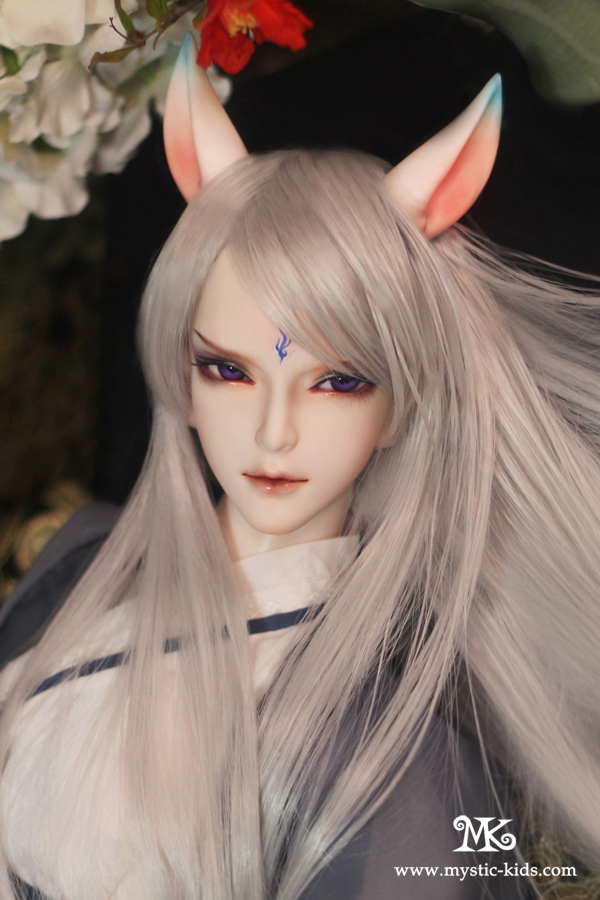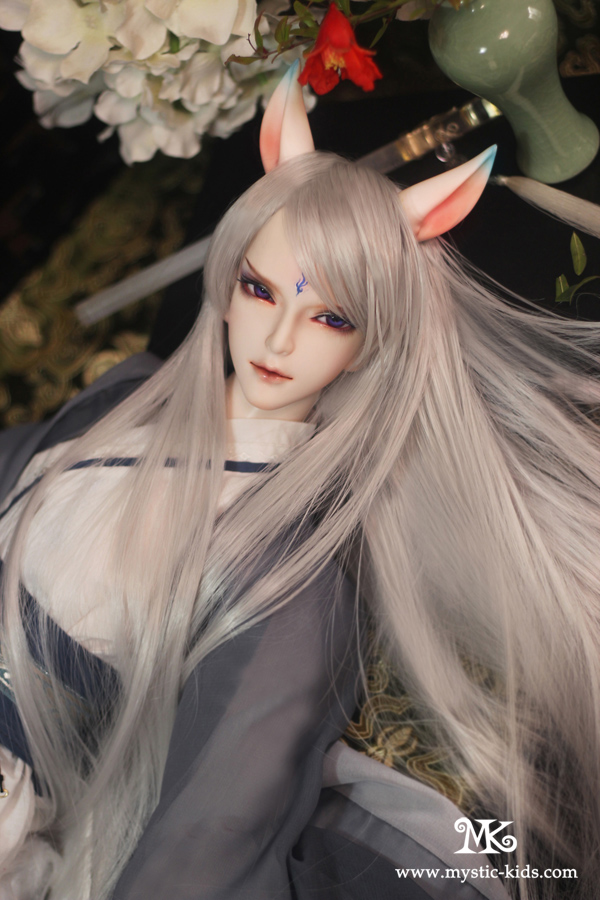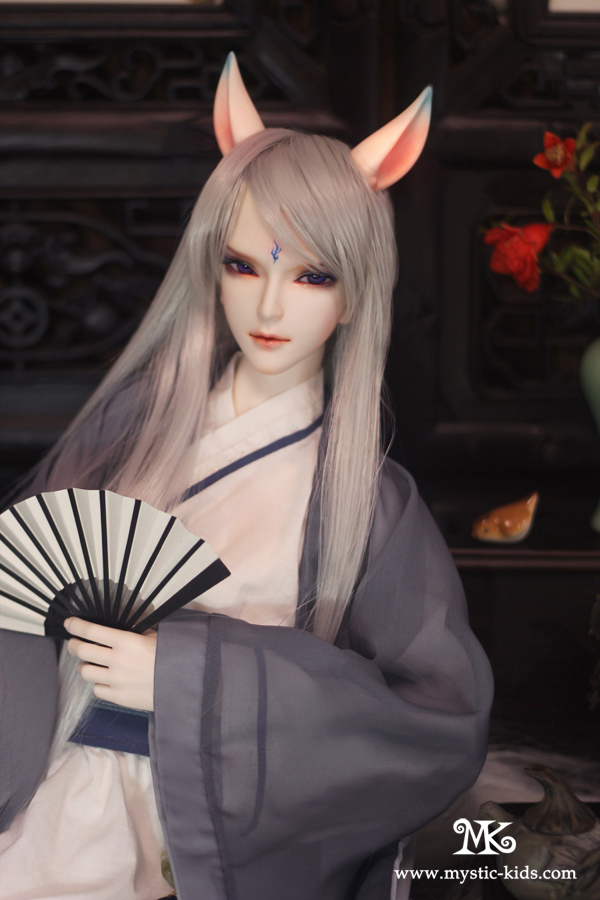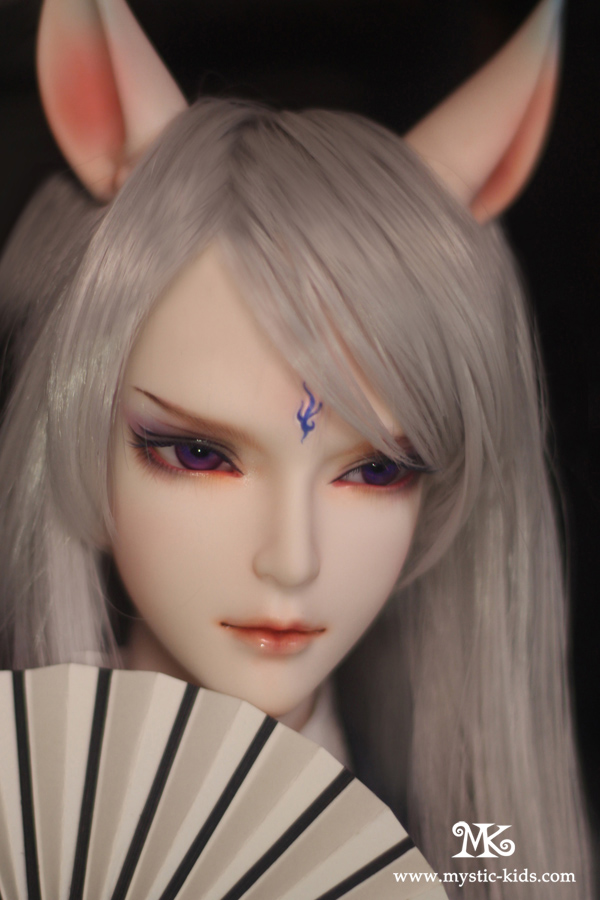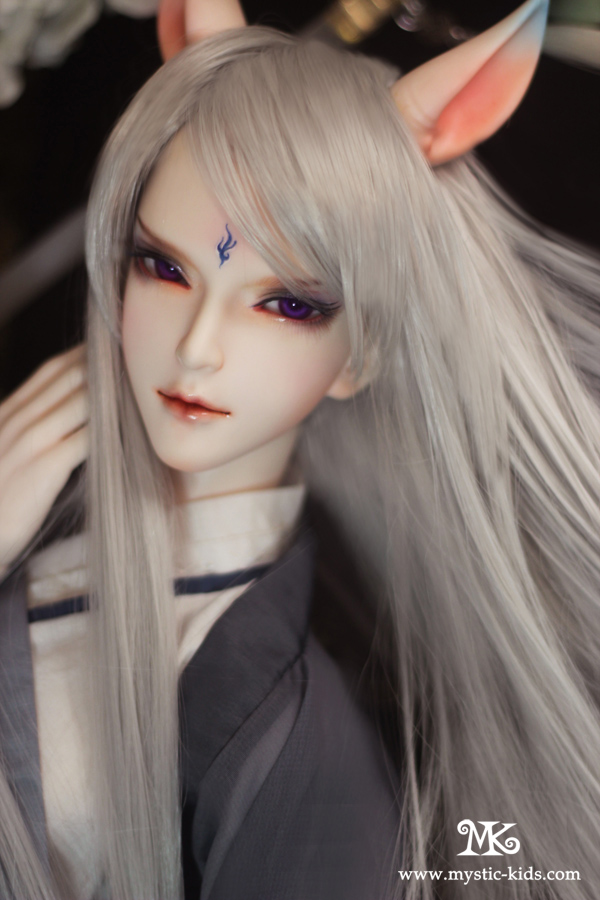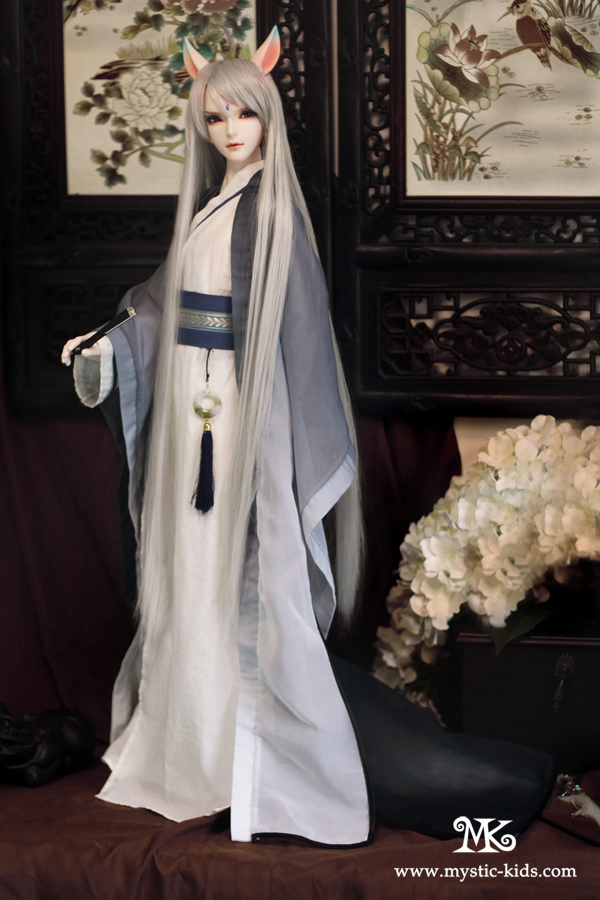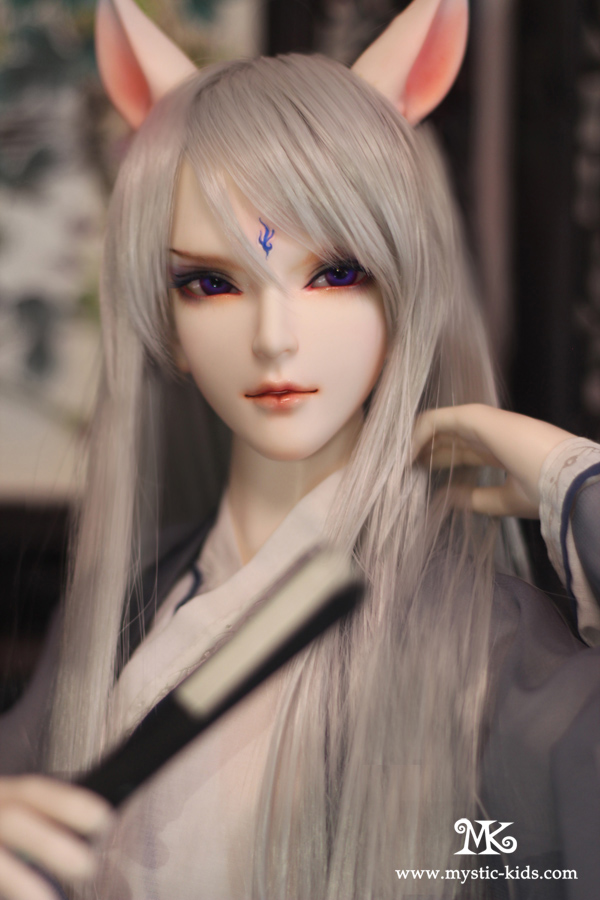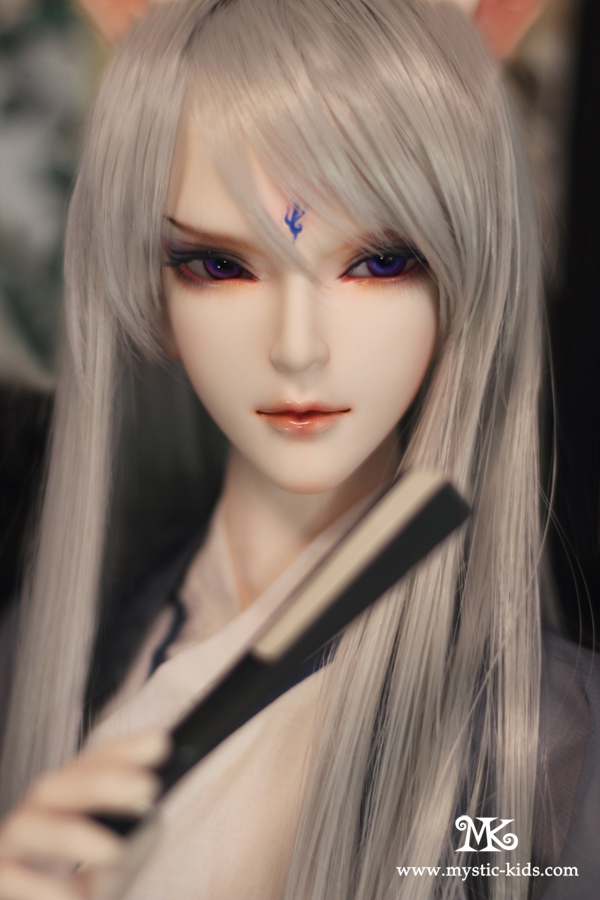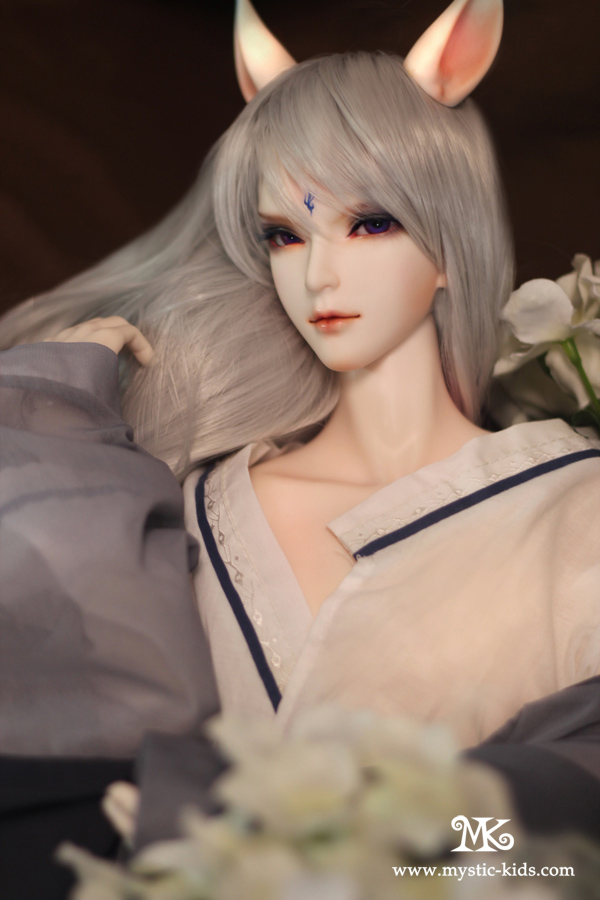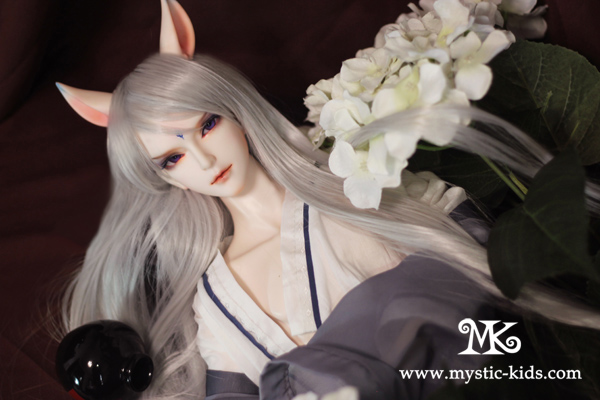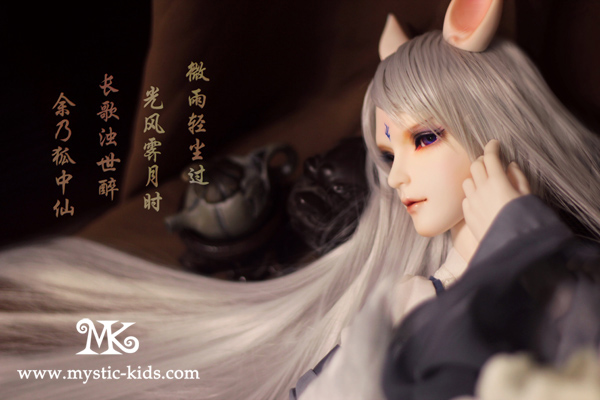MK71男娃身体尺寸